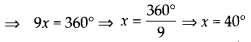# MP Board Class 8th Maths Solutions Chapter 3 Understanding Quadrilaterals Ex 3.2

## MP Board Class 8th Maths Solutions Chapter 3 Understanding Quadrilaterals Ex 3.2

Question 1.
Find x in the following figures.Solution:
(a) Sum of the measures of the exterior angles of any polygon is 360°.
∴ 125° + 125° + x = 360°
⇒ 250° + x = 360°
⇒ x = 360° – 250° ⇒ x = 110°.
(b) Since, sum of the exterior angles of any polygon is 360°.
∴ x + 90° + 60° + 90° + 70° = 360°
⇒ x + 310° = 360° => x = 360° – 310°
∴ x = 50°.Question 2.
Find the measure of each exterior angle of a regular polygon of
(i) 9 sides
(ii) 15 sides.
Solution:
(i) Let each exterior angle of a regular polygon who has 9 sides is equal to x.
Sum of exterior angles of any polygon is 360°.Thus each exterior angle of a regular polygon of 9 sides is 40°.
(ii) Let each exterior angle of a regular polygon who has 15 sides is x.
Sum of all exterior angles of a polygon is 360°.Thus each exterior angle of a regular polygon of 15 sides is 24°.Question 3.
How many sides does a regular polygon have if the measure of an exterior angle is 24°?
Solution:
Total measure of all exterior angles = 360°
Measure of each exterior angle = 24°
Therefore, the number of sides = $$\frac{360^{\circ}}{24^{\circ}}$$
= 15
The polygon has 15 sides.

Question 4.
How many sides does a regular polygon have if each of its interior angles is 165°?
Solution:
Total measure of all exterior angles = 360°
Measure of each interior angle = 165°
Measure of each exterior angle = 180° – 165°
= 15°
Therefore, number of sides = $$\frac{360^{\circ}}{15^{\circ}}$$ = 24Question 5.
(a) Is it possible to have a regular polygon with measure of each exterior angle as 22°?
(b) Can it be an interior angle of a regular polygon? Why ?
Solution:
(a) No, because 22° is not a divisor of 360°.
(b) No, because each interior angle is 180° – 22° = 158°, which is not a divisor of 360°.

Question 6.
(a) What is the minimum interior angle possible for a regular polygon? Why?
(b) What is the maximum exterior angle possible for a regular polygon?
Solution:
(a) Since each angle of an equilateral triangle is 60°.
And equilateral triangle is a regular polygon.
∴ Minimum interior angle is 60° for a regular polygon.
(b) Since minimum interior angle of a regular polygon is 60°.
∴ Each exterior angle of a regular polygon = 180° – 60° = 120°.
∴ Possible maximum exterior angle of a regular polygon is 120°.